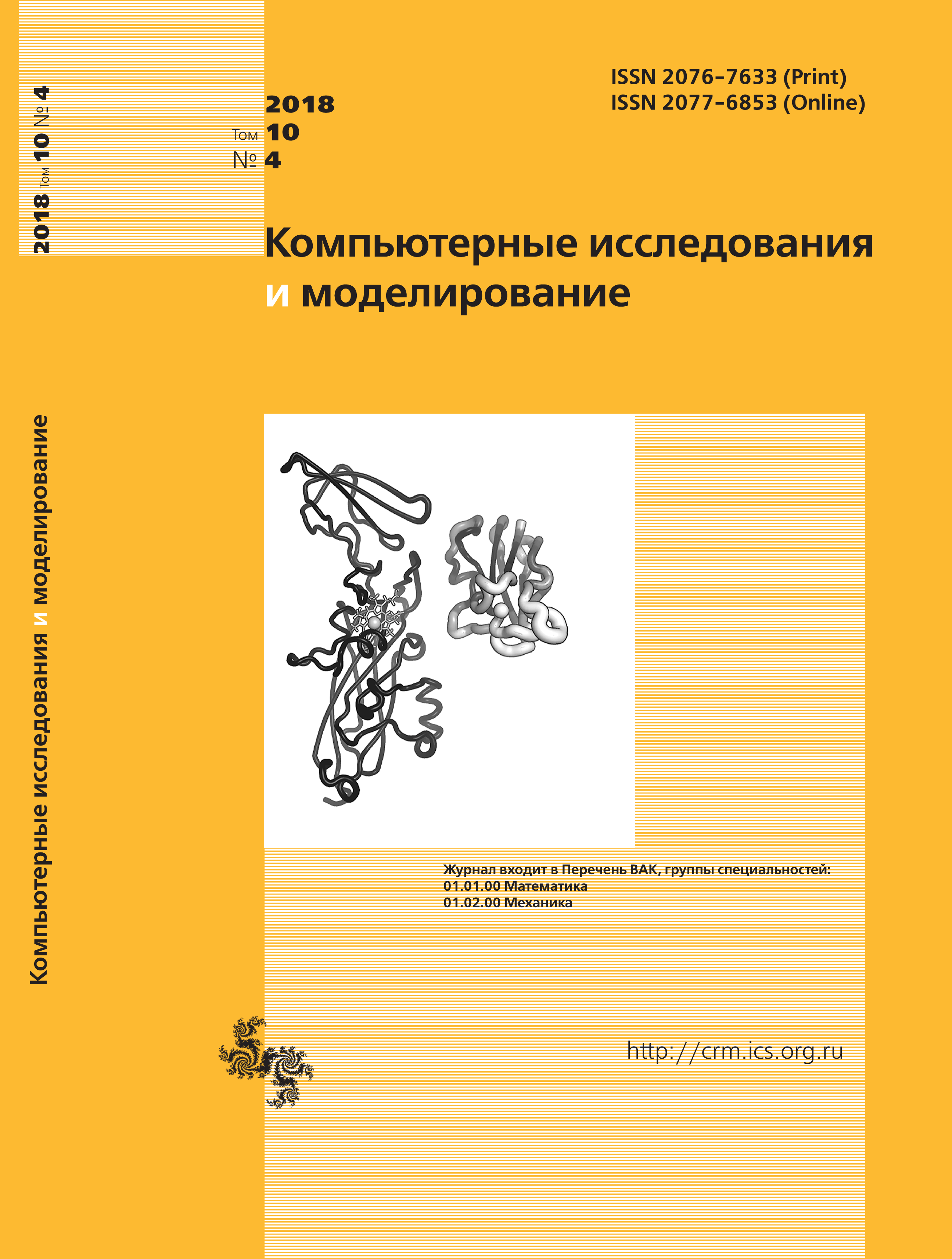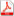Issue 4, 2018 Vol. 10

# All issues

Signal and noise calculation at Rician data analysis by means of combining maximum likelihood technique and method of momentspdf (352K)  / List of references

The paper develops a new mathematical method of the joint signal and noise calculation at the Rice statistical distribution based on combing the maximum likelihood method and the method of moments. The calculation of the sough-for values of signal and noise is implemented by processing the sampled measurements of the analyzed Rician signal’s amplitude. The explicit equations’ system has been obtained for required signal and noise parameters and the results of its numerical solution are provided confirming the efficiency of the proposed technique. It has been shown that solving the two-parameter task by means of the proposed technique does not lead to the increase of the volume of demanded calculative resources if compared with solving the task in one-parameter approximation. An analytical solution of the task has been obtained for the particular case of small value of the signal-to-noise ratio. The paper presents the investigation of the dependence of the sought for parameters estimation accuracy and dispersion on the quantity of measurements in experimental sample. According to the results of numerical experiments, the dispersion values of the estimated sought-for signal and noise parameters calculated by means of the proposed technique change in inverse proportion to the quantity of measurements in a sample. There has been implemented a comparison of the accuracy of the soughtfor Rician parameters’ estimation by means of the proposed technique and by earlier developed version of the method of moments. The problem having been considered in the paper is meaningful for the purposes of Rician data processing, in particular, at the systems of magnetic-resonance visualization, in devices of ultrasonic visualization, at optical signals’ analysis in range-measuring systems, at radar signals’ analysis, as well as at solving many other scientific and applied tasks that are adequately described by the Rice statistical model.

Keywords: probability density function, Rice distribution, maximum likelihood technique, method of moments, samples of measurements, signal to noise ratio
Citation in English: Yakovleva T.V. Signal and noise calculation at Rician data analysis by means of combining maximum likelihood technique and method of moments // Computer Research and Modeling, 2018, vol. 10, no. 4, pp. 511-523
Citation in English: Yakovleva T.V. Signal and noise calculation at Rician data analysis by means of combining maximum likelihood technique and method of moments // Computer Research and Modeling, 2018, vol. 10, no. 4, pp. 511-523
DOI: 10.20537/2076-7633-2018-10-4-511-523
Views (last year): 11.

Full-text version of the journal is also available on the web site of the scientific electronic library eLIBRARY.RU

The journal is included in the Russian Science Citation Index

The journal is included in the List of Russian peer-reviewed journals publishing the main research results of PhD and doctoral dissertations.

International Interdisciplinary Conference "Mathematics. Computing. Education"

The journal is included in the RSCI

Indexed in Scopus